# pylops.utils.wavelets.ricker#

pylops.utils.wavelets.ricker(t, f0=10, taper=None)[source]#

Ricker wavelet

Create a Ricker wavelet given time axis t and central frequency f_0

Parameters
tnumpy.ndarray

Time axis (positive part including zero sample)

f0float, optional

Central frequency

taperfunc, optional

Taper to apply to wavelet (must be a function that takes the size of the window as input

Returns
wnumpy.ndarray

Wavelet

tnumpy.ndarray

Symmetric time axis

wcenterint

Index of center of wavelet

## Examples using pylops.utils.wavelets.ricker#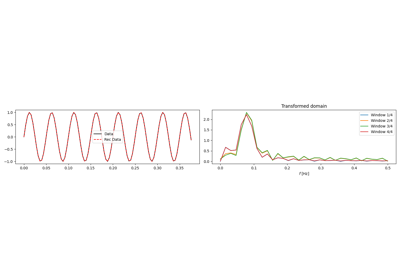1D, 2D and 3D Sliding

1D, 2D and 3D Sliding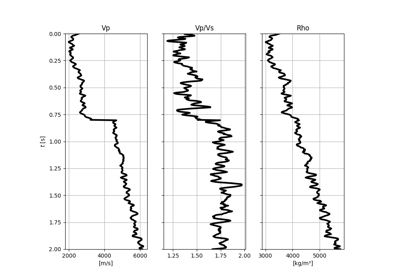AVO modelling

AVO modelling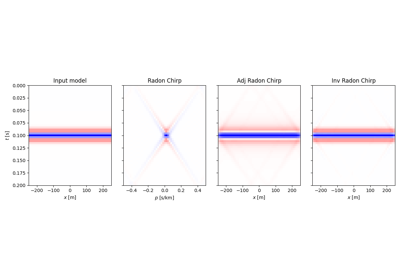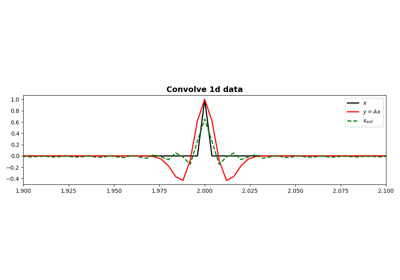Convolution

Convolution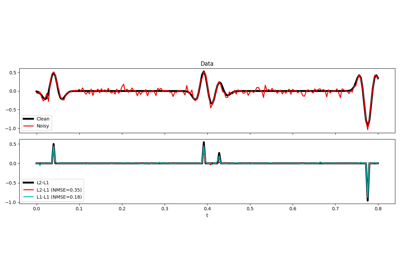L1-L1 IRLS

L1-L1 IRLS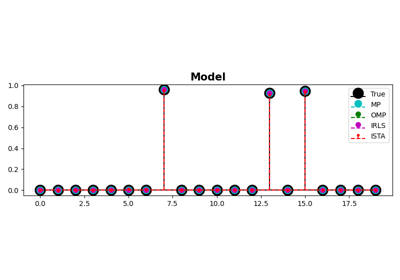MP, OMP, ISTA and FISTA

MP, OMP, ISTA and FISTA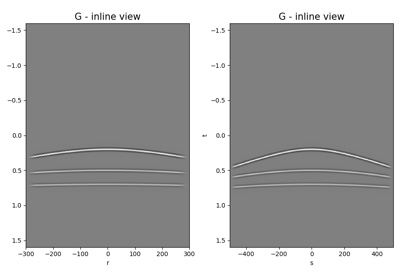Multi-Dimensional Convolution

Multi-Dimensional Convolution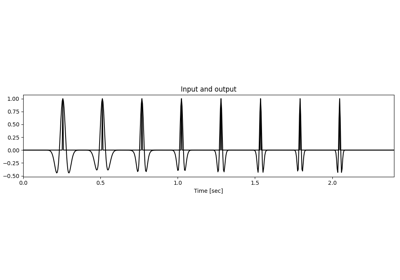Non-stationary Convolution

Non-stationary Convolution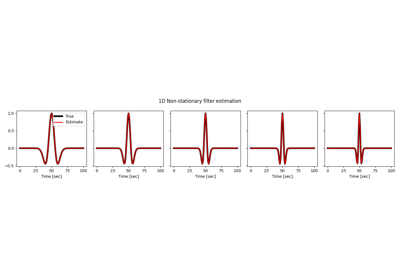Non-stationary Filter Estimation

Non-stationary Filter EstimationNormal Moveout (NMO) Correction

Normal Moveout (NMO) Correction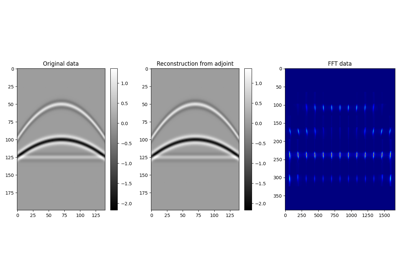Patching

Patching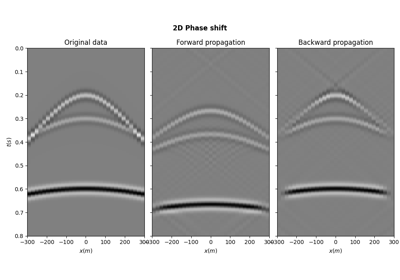PhaseShift operator

PhaseShift operator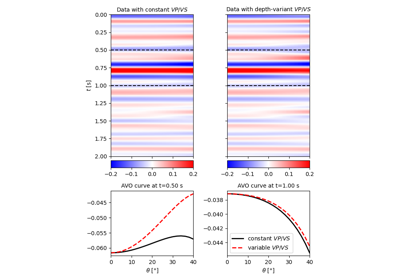Pre-stack modelling

Pre-stack modelling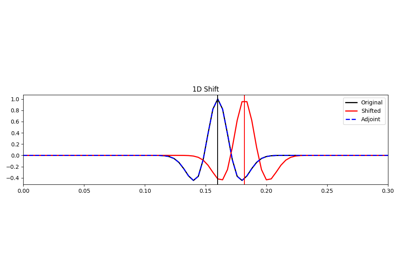Shift

Shift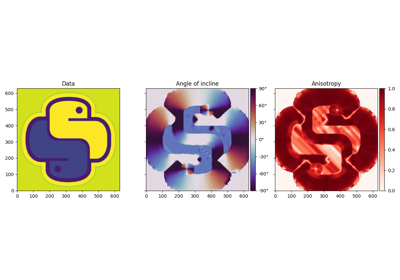Slope estimation via Structure Tensor algorithm

Slope estimation via Structure Tensor algorithm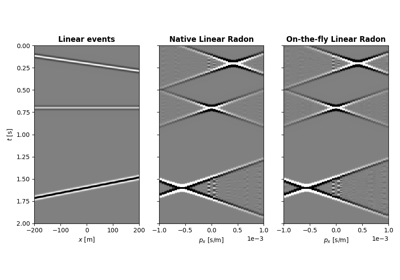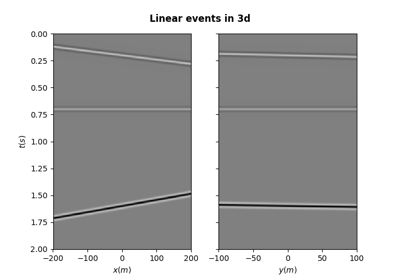Synthetic seismic

Synthetic seismic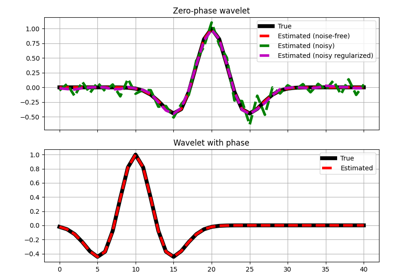Wavelet estimation

Wavelet estimation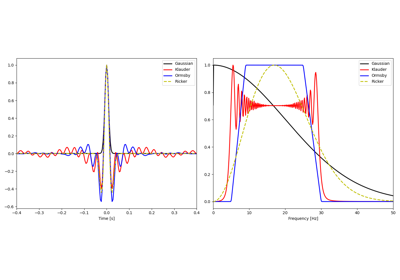Wavelets

Wavelets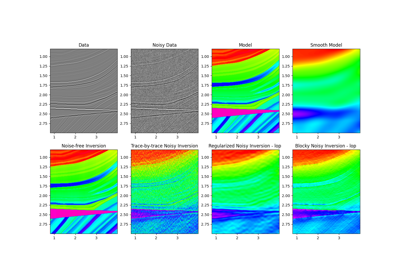07. Post-stack inversion

07. Post-stack inversion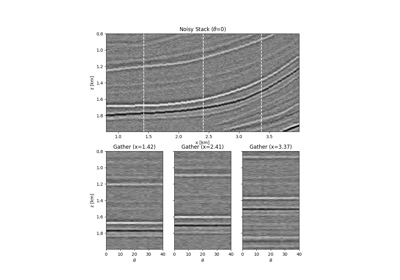08. Pre-stack (AVO) inversion

08. Pre-stack (AVO) inversion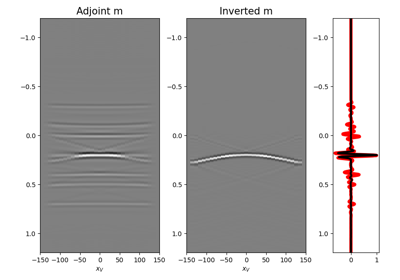09. Multi-Dimensional Deconvolution

09. Multi-Dimensional Deconvolution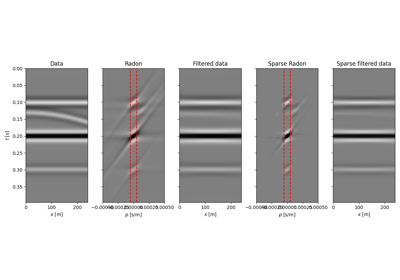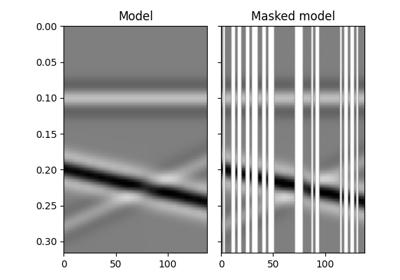12. Seismic regularization

12. Seismic regularization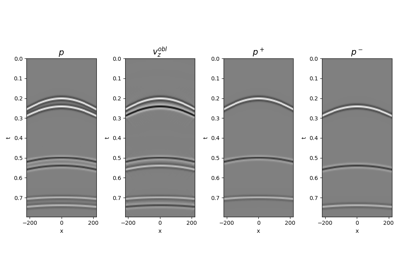14. Seismic wavefield decomposition

14. Seismic wavefield decomposition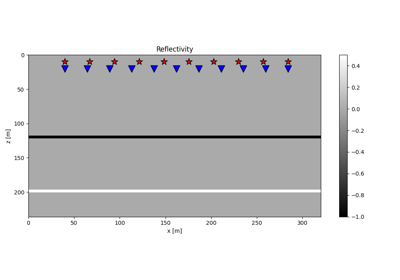15. Least-squares migration

15. Least-squares migration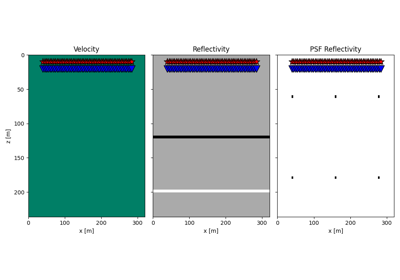20. Image Domain Least-squares migration

20. Image Domain Least-squares migration